Author: Oscar Cronquist Article last updated on May 05, 2022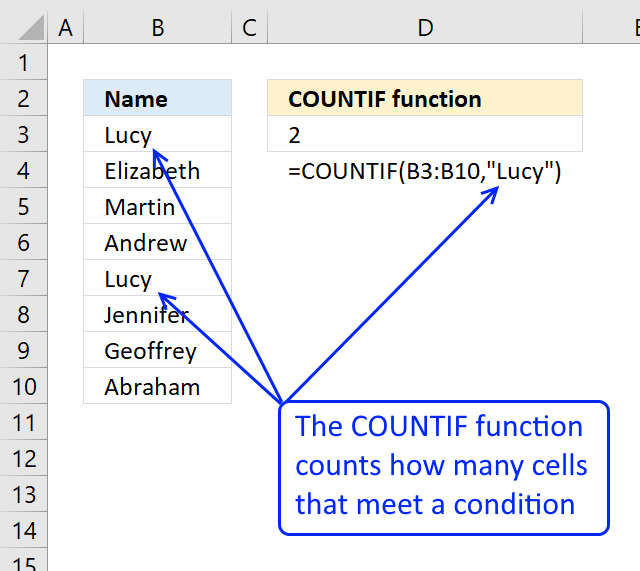The COUNTIF function calculates the number of cells that meet a given condition.

Formula in cell D3:

=COUNTIF(B3:B10,"Lucy")

Lucy is found twice, in cell B3 and B7, the function returns 2 in cell D3.

The condition is not case sensitive meaning condition "lucy" will also return 2 demonstrated in the image above.

Note that you can use multiple conditions in the second argument, however, you need to enter the formula as an array formula.

## 1. COUNTIF Function Syntax

COUNTIF(range, criteria)

## 2. COUNTIF Function Arguments

 range Required. The cell range you want to count the cells meeting a condition. criteria Required. The condition that you want to count.

## 3. How to count cells equal to a condition?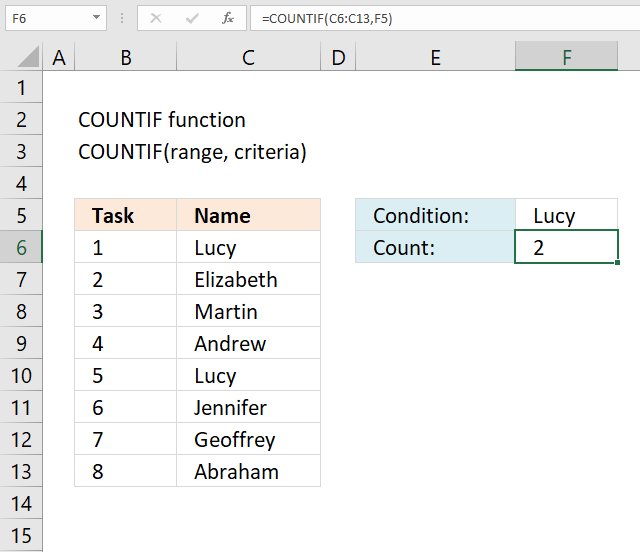The following formula in cell F6 counts the number of cells within cell range C6:C13 that equals the condition specified in cell F5.

=COUNTIF(C6:C13, F5)

You can also use a value instead of a cell reference inside the formula.

=COUNTIF(C6:C13, "Lucy")

Name "Lucy" is hardcoded into the formula in this example. Hardcoded values mean that the formula contains literal values and cell references are not being used.

The downside is that you need to change the formula if you need to use another value.

Cell reference C6:C13 is a relative cell reference meaning it will change if you copy the cell (not the formula) and paste it to other cells. Add dollar signs, to prevent this behavior, which will lock the cell reference. Example, \$C\$6:\$C\$13.

How to use absolute and relative references

COUNTIF(C6:C13, "Lucy")

becomes

COUNTIF({"Lucy"; "Elizabeth"; "Martin"; "Andrew"; "Lucy"; "Jennifer"; "Geoffrey"; "Abraham"}, "Lucy")

Note that the array has semicolons as a separating character, which shows that the values are located on a row each.

You can easily convert a cell reference to hardcoded values, select the cell range and then press function key F9. That will instantly convert the cell range to constants.

COUNTIF({"Lucy"; "Elizabeth"; "Martin"; "Andrew"; "Lucy"; "Jennifer"; "Geoffrey"; "Abraham"}, "Lucy")

returns 2 in cell D5. I have bolded the matching values to show that the correct value is 2.

## 4. Example 2 - Count cells larger/less than a criterion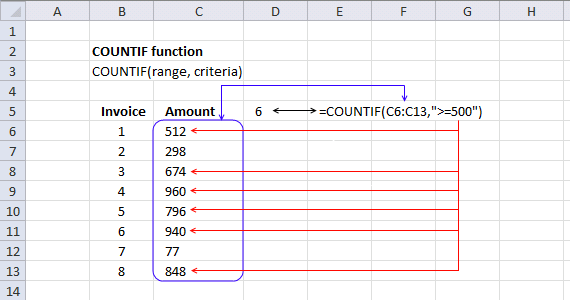The following formula in cell D5 counts the number of cells within cell range C6:C13 that is larger than or equal to 500. The image above has six numbers in cell range C6:C14 that are larger than or equal to 500.

The formula in cell D5 returns 6, the following six numbers 512, 674, 960, 796, 940 and 848 are larger than 500.

=COUNTIF(C6:C13, ">=500")

You can use these operators:

• < less than
• > larger than
• = equal sign
• <= less than or equal to
• >=larger than or equal to
• <> not equal to

Remember to use double quotes when you combine a number with an operator.

## 5. Example 3 - Count cells containing a text stringThe following formula in cell D5 counts the number of cells within cell range C6:C13 that contains the text string "apple":

=COUNTIF(C6:C13, "*apple*")

The asterisk matches no characters, any single character or any multiple characters. That is why "*apple*" matches "Orange, Apple", note also that the COUNTIF function is not taking into account upper and lower letters.

There is one more wildcard character you can use which is the question mark. The question mark allows you to match any single character.

=COUNTIF(C7:C13,"*appl?")

The formula above utilizes this condition "*appl?" and matches two cells in cell range C6:C13, displayed in the above image. They are "Orange, Apple" and "Kiwi, Pineapple".

## 6. Example 4 - Absolute and relative cell referenceWith clever use of absolute and relative cell references you can build formulas containing cell references that expand automatically when you copy the cell and paste to cells below.

Formula in cell C6:

=COUNTIF(\$B\$6:B6,B6)

\$B\$6:B6 is a cell reference to cell B6. When the cell is copied to cells below, the cell reference changes. The first part \$B\$6 i always locked to cell B6, the last part B6 changes. Cell range \$B\$6:B6 "grows" when you copy the cell.

This technique is used in this popular post: Filter unique distinct values

In cell C20:

=COUNTIF(\$B\$6:B20,B20)

becomes

=COUNTIF({"Watermelon"; "Banana"; "Orange"; "Kiwi"; "Lemon"; "Apple"; "Apricot"; "Banana"; "Pear"; "Apple"; "Pineapple"; "Banana"; "Pear"; "Pear"; "plum"},"plum") and returns 1 in cell C20

## 7. Example 5 - Array formulaArray formula in cell range C6:C20:

=COUNTIF(B6:B20, B6:B20)

### 7.1 How to enter an array formula

1.  Select cell range C6:C20
2. Copy / Paste formula to formula bar3. Press and hold CTRL + SHIFT
4. Press Enter
5. Release all keys
Note, Office 365 subscribers can now use dynamic arrays instead of regular array formulas.

### 7.2 Explaining the array formula

The COUNTIF function counts the number of cells within a range that meet a single criterion. In this example, I am using multiple values in the criteria argument.

Each value is in the criteria argument is used as a criterion and the returning array has the same number of values as the criteria argument.

The technique described here is used in this popular post: Count unique distinct values

=COUNTIF(B6:B20, B6:B20)

becomes

=COUNTIF({"Watermelon"; "Banana"; "Orange"; "Kiwi"; "Lemon"; "Apple"; "Apricot"; "Banana"; "Pear"; "Apple"; "Pineapple"; "Banana"; "Pear"; "Pear"; "plum"},{"Watermelon"; "Banana"; "Orange"; "Kiwi"; "Lemon"; "Apple"; "Apricot"; "Banana"; "Pear"; "Apple"; "Pineapple"; "Banana"; "Pear"; "Pear"; "plum"})

and returns {1; 3; 1; 1; 1; 2; 1; 3; 3; 2; 1; 3; 3; 3; 1} in cell range C6:C20.

## 8. How to count cells containing x number of characters?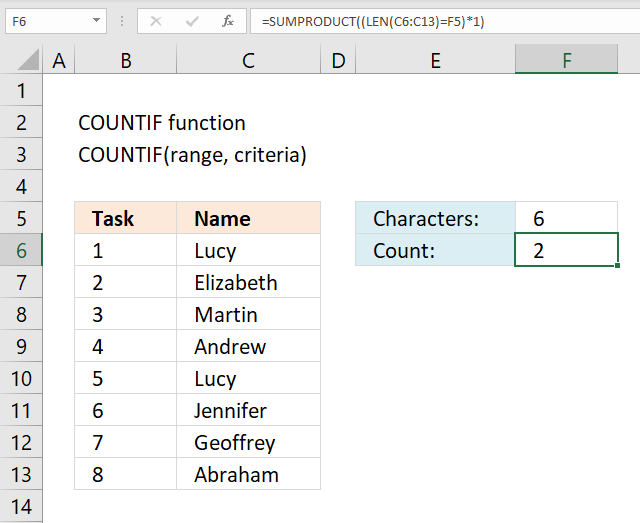Here is one downside with the COUNTIF function, you can't use other functions in the range argument. There are rare exceptions, one is the OFFSET function.

The following formula won't work in cell F6:

=COUNTIF(LEN(C6:C13), F5)

Excel shows a dialog box containing an error message.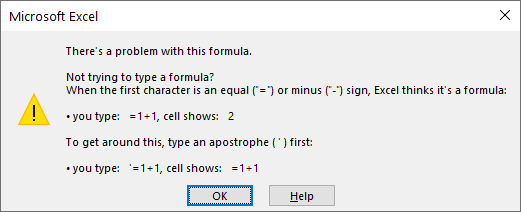The following formula works:

=SUMPRODUCT((LEN(C6:C13)=F5)*1)

### 8.1 Explaining formula in cell F6

#### Step 1 - Count characters for each cell

The LEN function counts the number of characters in a cell, the LEN function returns an array of numbers if you use a cell range.

LEN(C6:C13)

becomes

LEN({"Lucy"; "Elizabeth"; "Martin"; "Andrew"; "Lucy"; "Jennifer"; "Geoffrey"; "Abraham"})

and returns {4; 9; 6; 6; 4; 8; 8; 7}.

#### Step 2 - Compare with the number in cell F5

The equal sign allows you to compare values. We want to identify cells that have the same number of characters as the specified number in cell F5.

The logical expression returns TRUE or FALSE.

LEN(C6:C13)=F5

becomes

{4; 9; 6; 6; 4; 8; 8; 7}=F5

becomes

{4; 9; 6; 6; 4; 8; 8; 7}=6

and returns

{FALSE; FALSE; TRUE; TRUE; FALSE; FALSE; FALSE; FALSE}

#### Step 3 - Multiply with 1

The SUMPRODUCT can't sum boolean values, we need to convert them to their numerical equivalents. TRUE = 1, FALSE = 0 (zero).

(LEN(C6:C13)=F5)*1

becomes

{FALSE; FALSE; TRUE; TRUE; FALSE; FALSE; FALSE; FALSE}*1

and returns

{0;0;1;1;0;0;0;0}.

#### Step 4 - Sum numbers

The SUMPRODUCT function is able to add numbers in an array without the need to enter the formula as an array formula.

The formula becomes slightly larger compared to using the SUM function.

SUMPRODUCT((LEN(C6:C13)=F5)*1)

## 9. Dynamic array formula (Excel 365)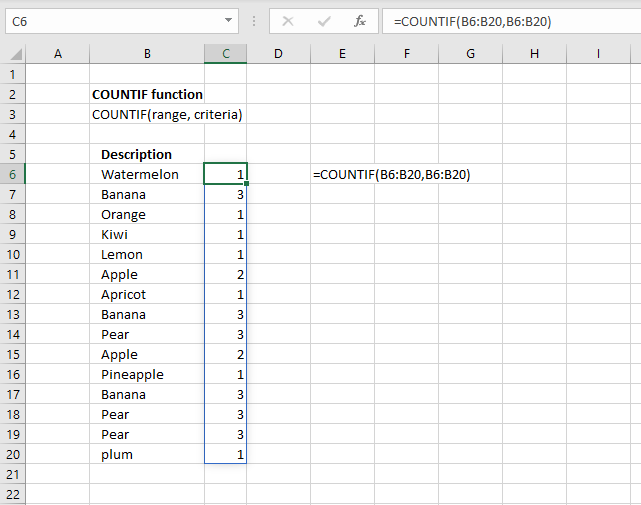You no longer need to enter the formula as an array formula if you are a subscriber of Office 365, the new feature is called "Dynamic Arrays" and was introduced to Excel in January 2020.

It will automatically detect if a formula returns more than one value and will extend accordingly based on the number of values that are being returned, this is called spilling.

The image above demonstrates this behavior, the formula has extended automatically to cells below as far as needed. A blue border indicates that spilling has occurred, however, it will disappear as soon as you press with left mouse button on outside the formula range.